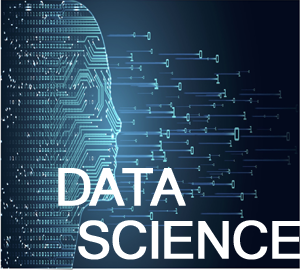# Data Science Training in Hyderabad | Data Science Course in Kukatpally/KPHB | Data Science Online Training

##• Over view of Data Science concepts
• Introduction Software tools & library’s etc…
• Jupyter Notebook /Spyder
• Python / R / Matlab Introduction & simple programming
• Loops with programming
• Functions & Class functions generation
• Import functions & generate user define import functions
• Introduction to Numpy Basics and utilization
• Introduction to SciPy - Linear Algebra and utilization
• Introduction to Pandas Basics and utilization
• Introduction to Scikit-Learn Basics and utilization
• Introduction to Matplotlib Basics and utilization
• Introduction to Seaborn Basics and utilization
• Introduction to Bokeh Basics and utilization
• Introduction to GUI & Graphics Basics and utilization

Assessment Test

• Introduction to Algorithm and how it is implement
• Algorithm_1 Linear Regression
• Algorithm_2 Logistic Regression
• Algorithm_3 Decision Trees
• Algorithm_4 Cart (Classification and Regression Tree)
• Algorithm_5 Naive Bayes
• Algorithm_6 KNN (K-Nearest Neighbors)
• Algorithm_7 Support Vector Machine (SVM)
• Algorithm_8 Principal Component Analysis (PCA)
• Mini project Starts
• Project Literature Survey work
• Project Motivation
• Doubts Clarification
• Main project work